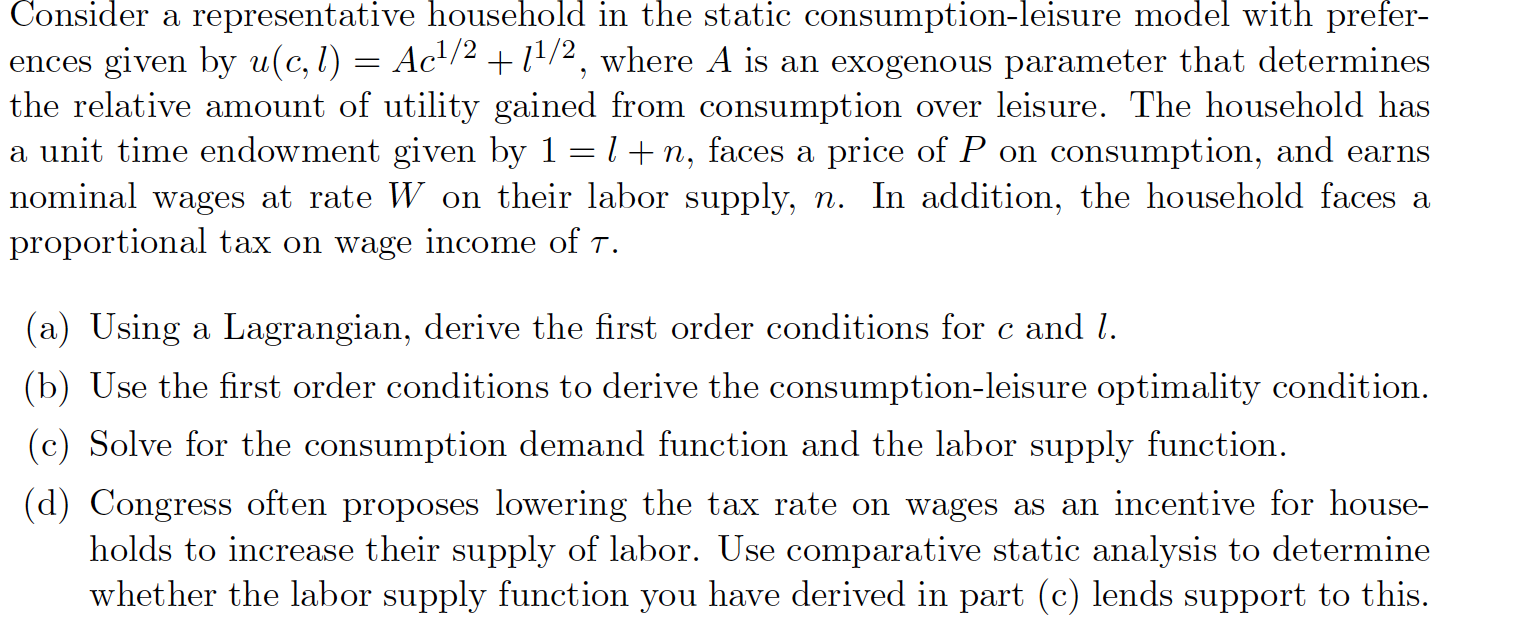### Create an Account

Home / Questions / Consider a representative household in the static consumption-leisure model with prefer- e...

# Consider a representative household in the static consumption-leisure model with prefer- ences given by u(c,l) = Ac1/2 +11/2, where A is an exogenous parameter that determines the relative amount of

Consider a representative household in the static consumption-leisure model with prefer- ences given by u(c,l) = Ac1/2 +11/2, where A is an exogenous parameter that determines the relative amount of utility gained from consumption over leisure. The household has a unit time endowment given by 1 =1+n, faces a price of P on consumption, and earns nominal wages at rate W on their labor supply, n. In addition, the household faces a proportional tax on wage income of T. (a) Using a Lagrangian, derive the first order conditions for c and 1. (b) Use the first order conditions to derive the consumption-leisure optimality condition. (c) Solve for the consumption demand function and the labor supply function. (d) Congress often proposes lowering the tax rate on wages as an incentive for house- holds to increase their supply of labor. Use comparative static analysis to determine whether the labor supply function you have derived in part (c) lends support to this.Jun 01 2021 View more View LessSubscribe To Get Solution